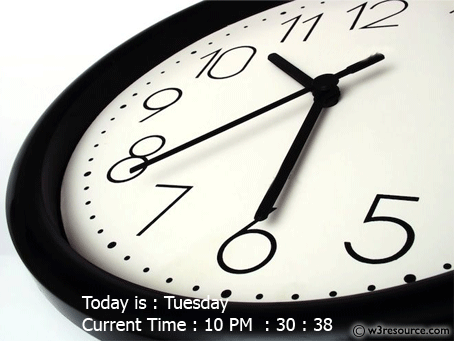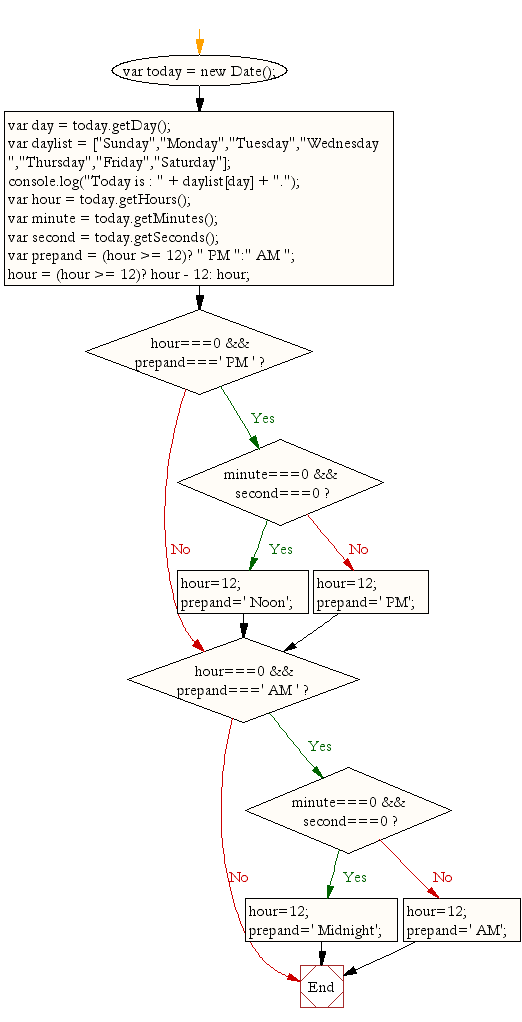# JavaScript: Display the current day and time in a specific format

## JavaScript Basic: Exercise-1 with Solution

Write a JavaScript program to display the current day and time in the following format.
Today is : Tuesday.
Current time is : 10 PM : 30 : 38

Pictorial Presentation:Sample Solution:

HTML Code:

``````<!DOCTYPE html>
<html>
<meta charset="utf-8">
<title>JavaScript current day and time</title>
<body></body>
</html>
```
```

JavaScript Code:

``````var today = new Date();
var day = today.getDay();
var daylist = ["Sunday","Monday","Tuesday","Wednesday ","Thursday","Friday","Saturday"];
console.log("Today is : " + daylist[day] + ".");
var hour = today.getHours();
var minute = today.getMinutes();
var second = today.getSeconds();
var prepand = (hour >= 12)? " PM ":" AM ";
hour = (hour >= 12)? hour - 12: hour;
if (hour===0 && prepand===' PM ')
{
if (minute===0 && second===0)
{
hour=12;
prepand=' Noon';
}
else
{
hour=12;
prepand=' PM';
}
}
if (hour===0 && prepand===' AM ')
{
if (minute===0 && second===0)
{
hour=12;
prepand=' Midnight';
}
else
{
hour=12;
prepand=' AM';
}
}
console.log("Current Time : "+hour + prepand + " : " + minute + " : " + second);
```
```

Sample Output:

```Today is : Tuesday.
Current Time : 10 PM  : 30 : 38
```

Explanations:

Declaring a JavaScript date : In JavaScript Date objects are based on a time value that is the number of milliseconds since 1 January, 1970 UTC. You can declare a date in the following ways :

```new Date();
new Date(value);
new Date(dateString);
new Date(year, month[, day[, hour[, minutes[, seconds[, milliseconds]]]]]);
```

The getDay() method is used to get the day of the week for the specified date according to local time, where 0 represents Sunday. The value returned by getDay() is an integer corresponding to the day of the week: 0 for Sunday, 1 for Monday, 2 for Tuesday, and so on.

The getHours() method is used to get the hour for a given date, according to local time. The value returned by getHours() is an integer between 0 and 23.

The getMinutes() method is used to get the minutes in the specified date according to local time. The value returned by getMinutes() is an integer between 0 and 59.

The getSeconds() method is used to get the seconds in the specified date according to local time. The value returned by getSeconds() is an integer between 0 and 59.

AM and PM : AM stands for 'ante meridiem', which means 'before noon' in Latin, while PM stands for 'post meridiem', which means 'after noon' in Latin.
12-Hour Periods : Nowadays most clocks are 12-hour clocks â€“ they divide the 24 hours in a day into two 12-hour periods.
Ante meridiem: Before noon - Between midnight (0:00) & noon (12:00)
Post meridiem: After noon Between noon (12:00) & midnight (0:00)

Flowchart:ES6 Version:

``````const today = new Date();
const day = today.getDay();
const daylist = ["Sunday","Monday","Tuesday","Wednesday ","Thursday","Friday","Saturday"];
console.log(`Today is : \${daylist[day]}.`);
let hour = today.getHours();
const minute = today.getMinutes();
const second = today.getSeconds();
let prepand = (hour >= 12)? " PM ":" AM ";
hour = (hour >= 12)? hour - 12: hour;
if (hour===0 && prepand===' PM ')
{
if (minute===0 && second===0)
{
hour=12;
prepand=' Noon';
}
else
{
hour=12;
prepand=' PM';
}
}
if (hour===0 && prepand===' AM ')
{
if (minute===0 && second===0)
{
hour=12;
prepand=' Midnight';
}
else
{
hour=12;
prepand=' AM';
}
}
console.log(`Current Time : \${hour}\${prepand} : \${minute} : \${second}`);

``````

Live Demo:

See the Pen JavaScript current day and time - basic-ex-2 by w3resource (@w3resource) on CodePen.

Improve this sample solution and post your code through Disqus

What is the difficulty level of this exercise?

Test your Programming skills with w3resource's quiz.

﻿

## JavaScript: Tips of the Day

Randomizes the order of the values of an array, returning a new array

Example:

```const tips_shuffle = ([...arr]) => {
let x = arr.length;
while (x) {
const i = Math.floor(Math.random() * x--);
[arr[x], arr[i]] = [arr[i], arr[x]];
}
return arr;
};
const foo = [2, 4, 6];
console.log(tips_shuffle(foo));
```

Output:

```[4, 2, 6]
```

We are closing our Disqus commenting system for some maintenanace issues. You may write to us at reach[at]yahoo[dot]com or visit us at Facebook